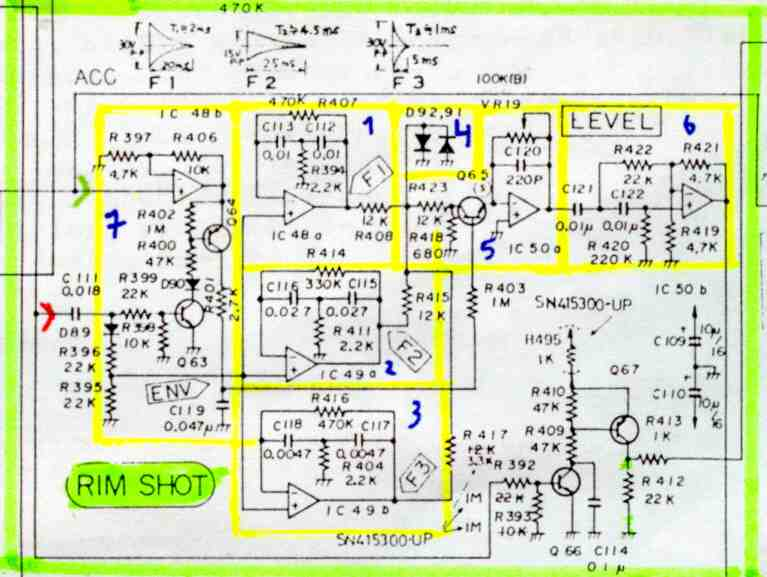Rim Shot> = Trig > = Accent or Velocity (from D/A) > = Noise

The Rimshot is the easiest instrument on this machine. It's based on three bridged T-network-filters (see parts 1,2,3). These kind of bandpass filter is the base for nearly every sound on the TR-808 (except HiHat and Cymbal).

They have different Q- and f-values as you can see on the diagrams above the schematic. According to these you'll get following frequencies : f1=500 Hz, f2=220 Hz, f3=1000Hz. The disadvantage of this circuit is, that frequency depends on every resistor (5% types used) and every capacitor. Every fault sums up by principles of superposition and can cause large differences between the same sound from machine to machine. That's perhaps the reason why Roland used VCO's for the other drumsounds of the 909.

When TRIG (>) is applied, oscillators 1,2,3 get a positive pulse from D89 and start oscillation. The decay time depends on Q-factor which can be calculated by the following equation: Q=(sqrt(R1/R2))/(sqrt(C1/C2)+sqrt(C2/C1)).

The outputs are mixed and clipped by a simple diode-clipper (part 4) D91,92. The clipped signal passes through a VCA (part 5) which gets control voltage from IC48b, Q64 and a simple envelope C119 (see part 7). Last stage is a high pass filter (part 6) which removes unwanted low frequencies to provide a more realistic simulation of the instrument.

This site is NOT optimized for a certain crap